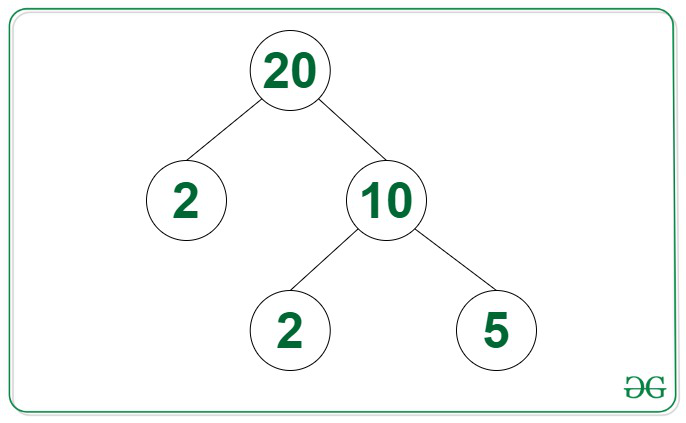# Height of Factor Tree for a given number

• Difficulty Level : Medium
• Last Updated : 21 Sep, 2021

Given a positive integer N, the task is to find the height of the Factor Tree of the given integer N.

Examples:

Input: N = 20
Output: 3
Explaination: The height of the Factor Tree of 20 shown in the image below is 3.Input: N = 48
Output: 5

Approach: The given problem can be solved by using the steps discussed below:

• Initialize a variable, say height as 0 that stores the height of the Factor Tree of the given integer N.
• Iterate over all the values of i in the range [2, √N] and perform the following steps:
1. Find the smallest divisor of N and if it exists, then increment the value of height by 1.
2. If a divisor i exists, then repeat the above step by updating the value of N to N / i, until N > 1.
3. If no divisors of N exist break the loop.
• After completing the above steps, print the value of height as the answer.

Below is the implementation of the above approach:

## C++

 `// C++ program for the above approach` `#include ``using` `namespace` `std;` `// Function to find the height of the``// Factor Tree of the integer N``int` `factorTree(``int` `N)``{``    ``// Stores the height of Factor Tree``    ``int` `height = 0;` `    ``// Loop to iterate over values of N``    ``while` `(N > 1) {``        ``// Stores if there exist``        ``// a factor of N or not``        ``bool` `flag = ``false``;` `        ``// Loop to find the smallest``        ``// factor of N``        ``for` `(``int` `i = 2; i <= ``sqrt``(N); i++) {``            ``// If i is a factor of N``            ``if` `(N % i == 0) {``                ``N = N / i;``                ``flag = ``true``;``                ``break``;``            ``}``        ``}` `        ``// Increment the height``        ``height++;` `        ``// If there are no factors of N``        ``// i.e, N is prime, break loop``        ``if` `(!flag) {``            ``break``;``        ``}``    ``}` `    ``// Return Answer``    ``return` `height;``}` `// Driver Code``int` `main()``{``    ``int` `N = 48;``    ``cout << factorTree(N);` `    ``return` `0;``}`

## Java

 `// Java program for the above approach``public` `class` `Main``{``    ``// Function to find the height of the``    ``// Factor Tree of the integer N``    ``static` `int` `factorTree(``int` `N)``    ``{``       ` `        ``// Stores the height of Factor Tree``        ``int` `height = ``0``;``      ` `        ``// Loop to iterate over values of N``        ``while` `(N > ``1``)``        ``{``          ` `            ``// Stores if there exist``            ``// a factor of N or not``            ``boolean` `flag = ``false``;``      ` `            ``// Loop to find the smallest``            ``// factor of N``            ``for` `(``int` `i = ``2``; i <= Math.sqrt(N); i++)``            ``{``              ` `                ``// If i is a factor of N``                ``if` `(N % i == ``0``) {``                    ``N = N / i;``                    ``flag = ``true``;``                    ``break``;``                ``}``            ``}``      ` `            ``// Increment the height``            ``height++;``      ` `            ``// If there are no factors of N``            ``// i.e, N is prime, break loop``            ``if` `(!flag) {``                ``break``;``            ``}``        ``}``      ` `        ``// Return Answer``        ``return` `height;``    ``}``    ` `    ``public` `static` `void` `main(String[] args) {``        ``int` `N = ``48``;``        ``System.out.println(factorTree(N));``    ``}``}` `// This code is contributed by mukesh07.`

## Python3

 `# Python 3 program for the above approach``from` `math ``import` `sqrt` `# Function to find the height of the``# Factor Tree of the integer N``def` `factorTree(N):``  ` `    ``# Stores the height of Factor Tree``    ``height ``=` `0` `    ``# Loop to iterate over values of N``    ``while` `(N > ``1``):``      ` `        ``# Stores if there exist``        ``# a factor of N or not``        ``flag ``=` `False` `        ``# Loop to find the smallest``        ``# factor of N``        ``for` `i ``in` `range``(``2``,``int``(sqrt(N))``+``1``,``1``):``            ``# If i is a factor of N``            ``if` `(N ``%` `i ``=``=` `0``):``                ``N ``=` `N ``/``/` `i``                ``flag ``=` `True``                ``break` `        ``# Increment the height``        ``height ``+``=` `1` `        ``# If there are no factors of N``        ``# i.e, N is prime, break loop``        ``if` `(flag``=``=``False``):``            ``break` `    ``# Return Answer``    ``return` `height` `# Driver Code``if` `__name__ ``=``=` `'__main__'``:``    ``N ``=` `48``    ``print``(factorTree(N))``    ` `    ``# This code is contributed by SURENDRA_GANGWAR.`

## C#

 `// C# program for the above approach``using` `System;``class` `GFG {``    ` `    ``// Function to find the height of the``    ``// Factor Tree of the integer N``    ``static` `int` `factorTree(``int` `N)``    ``{``      ` `        ``// Stores the height of Factor Tree``        ``int` `height = 0;``     ` `        ``// Loop to iterate over values of N``        ``while` `(N > 1) {``            ``// Stores if there exist``            ``// a factor of N or not``            ``bool` `flag = ``false``;``     ` `            ``// Loop to find the smallest``            ``// factor of N``            ``for` `(``int` `i = 2; i <= Math.Sqrt(N); i++) {``                ``// If i is a factor of N``                ``if` `(N % i == 0) {``                    ``N = N / i;``                    ``flag = ``true``;``                    ``break``;``                ``}``            ``}``     ` `            ``// Increment the height``            ``height++;``     ` `            ``// If there are no factors of N``            ``// i.e, N is prime, break loop``            ``if` `(!flag) {``                ``break``;``            ``}``        ``}``     ` `        ``// Return Answer``        ``return` `height;``    ``}` `  ``static` `void` `Main() {``    ``int` `N = 48;``    ``Console.Write(factorTree(N));``  ``}``}` `// This code is contributed by decode2207.`

## Javascript

 ``
Output:
`5`

Time Complexity: O(√N*log N)
Auxiliary Space: O(1)

My Personal Notes arrow_drop_up